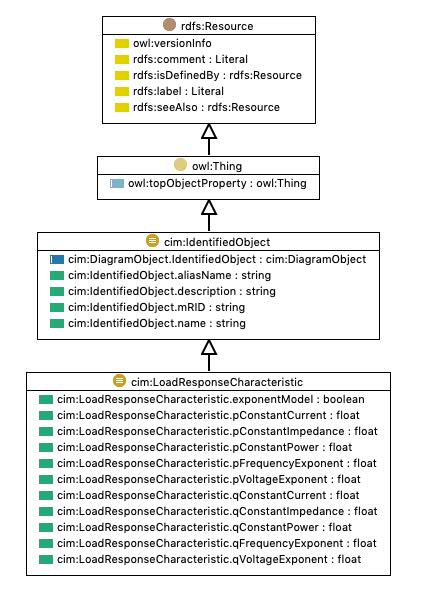type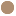Class [owl:Class]
comment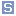Models the characteristic response of the load demand due to changes in system conditions such as voltage and frequency. This is not related to demand response. If LoadResponseCharacteristic.exponentModel is True, the voltage exponents are specified and used as to calculate: Active power component = Pnominal * (Voltage/cim:BaseVoltage.nominalVoltage) ** cim:LoadResponseCharacteristic.pVoltageExponent Reactive power component = Qnominal * (Voltage/cim:BaseVoltage.nominalVoltage)** cim:LoadResponseCharacteristic.qVoltageExponent Where * means "multiply" and ** is "raised to power of".
subClassOf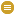cim:IdentifiedObject
equivalentClass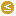cim:LoadResponseCharacteristic.qConstantImpedance max 1 floatcim:LoadResponseCharacteristic.qConstantPower max 1 floatcim:LoadResponseCharacteristic.pConstantPower max 1 floatcim:LoadResponseCharacteristic.qVoltageExponent max 1 floatcim:LoadResponseCharacteristic.pFrequencyExponent max 1 floatcim:LoadResponseCharacteristic.pConstantImpedance max 1 floatcim:LoadResponseCharacteristic.qConstantCurrent max 1 floatcim:LoadResponseCharacteristic.pVoltageExponent max 1 floatcim:LoadResponseCharacteristic.exponentModel max 1 booleancim:LoadResponseCharacteristic.pConstantCurrent max 1 floatcim:LoadResponseCharacteristic.qFrequencyExponent max 1 float

Generated with TopBraid Composer by TopQuadrant, Inc.